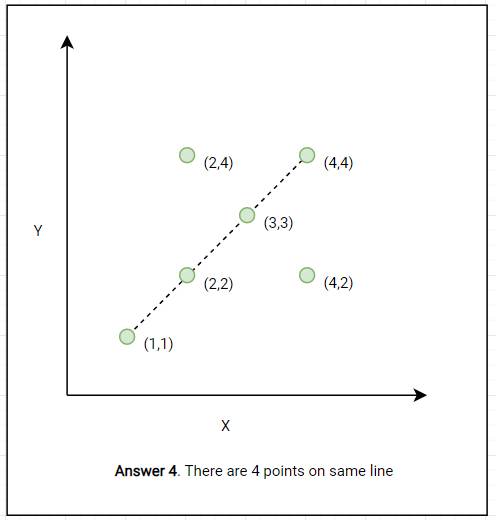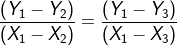## Max Points on a Line

Given n points on a 2D plane, find the maximum number of points that lie on the same straight line.

Example 1:We can always draw a straight using 2 points. But can we include the third point on it?

This can be solved by calculating the slope of the points. Three points `(X1,Y1)`,`(X2,Y2)` and `(X3,Y3)` are co-linear if slopes of lines with any two points are the same. The slope of 2 points can be calculated by the following formula:

```Point 1 = (X1,Y1)
Point 2 = (X2,Y2)

Slope = change in Y / change in X
Slope = (Y2-Y1) / (X2-X1)
```

So by applying the same formula three points `(X1,Y1)`,`(X2,Y2)` and `(X3,Y3)` are co-linear if :The Algorithm is:

1. Iterate over each pair of points.
2. Calculate the slope for each pair of points, say `Slope = X`/Y` = (X1 - X2 ) / (Y1 - Y2).`
3. Group all the points together which share the same slope value `X`/Y``. They are co-linear or in other words, each of these groups represents a straight line.
4. The group with maximum points is our answer. This line has a maximum number of points.
``````import java.util.HashMap;
import java.util.Map;

public class MaxPointInLine {

public static int maxPoints(int[][] points) {
if (points == null || points.length == 0) {
return 0;
}
if (points.length < 3) {
return points.length;
}
Map<Integer, Map<Integer, Integer>> pointCountBySlopeYBySlopeX = new HashMap<>();
int result = 0;
for (int i = 0; i < points.length; i++) {
//Reset map when considering for every point
pointCountBySlopeYBySlopeX.clear();
int x_1 = points[i];
int y_1 = points[i];

//Reset overlap and max declaration to 0 when
// considering for every fresh point
int max = 0;
int overlap = 0;
for (int j = i + 1; j < points.length; j++) {
int x_2 = points[j];
int y_2 = points[j];

//compute the slope numerator and denominator
int slope_x = (x_2 - x_1);
int slope_y = (y_2 - y_1);

//If both x and y == 0 then both the points in consideration are same.
// These can be duplicate or overlapping points.So increment overlap
//and continue the loop since there is no slope for overlapping or
// duplicate points
if (slope_x == 0 && slope_y == 0) {
overlap++;
continue;
}
//Compute the gcd of x and y; so that 2/4 is considered same as 1/2
int gcd = generateGCD(slope_x, slope_y);
if (gcd != 0) {
slope_x = slope_x / gcd;
slope_y = slope_y / gcd;
}

Map<Integer,Integer> pointCountBySlopeY = pointCountBySlopeYBySlopeX.get(slope_x);
if (pointCountBySlopeY == null) {
pointCountBySlopeY = new HashMap < > ();
pointCountBySlopeYBySlopeX.put(slope_x, pointCountBySlopeY);
}
Integer count = pointCountBySlopeY.get(slope_y);
if (count == null) {
count = 1;
pointCountBySlopeY.put(slope_y, count);
} else {
count = count + 1;
pointCountBySlopeY.put(slope_y, count);
}
//Local max
max = Math.max(max, count);
}
//global maximum
result = Math.max(max + overlap + 1, result);
}
return result;
}

private static int generateGCD(int a, int b) {
if (b == 0) return a;
else return generateGCD(b, a % b);
}

public static void main(String[] args) {
int[][] points = {{1, 1}, {3, 2}, {5, 3}, {4, 1}, {2, 3}, {1, 4}};
System.out.println(maxPoints(points));
}
}``````
• Time Complexity: `O(N2)`
• Space Complexity`O(N)` to track down not more than `N - 1` lines.
Arrays Algorithm